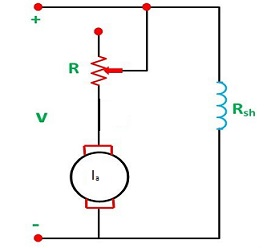## Saturday, 29 February 2020## Necessity of a Starter For D.C. Motor

Necessity of a Starter For D.C. Motor :

In any D.C. Motor, the back e.m.f. when the armature is stationary is equal to zero. The result is the motor ohmic resistance has to oppose the applied voltage. Since the resistance of the armature circuit is low, high amount of current is drawn from the supply to oppose the applied voltage without a starter, The magnitude of this current may exceed several times the rated current damaging the motor itself. Hence, to protect the armature, extra resistance is added in the armature circuit limiting the armature current to its rated value and is gradually decreased as back develops. To satisfy this condition extra variable resistance is connected in the armature circuit in a starter. A starter basically consists of resistance in series to the armature circuit such that it can be varied in steps and as the speed develops, for every step the back e.m.f. develops and the value of armature current is controlled.

Starter for D.C. Shunt Motor :

The back e.m.f. equation for a d.c. shunt motor is

Eb = V — laRa or K1ΦN = V — laRa

At the time of starting, the speed (N) is equal to zero and therefore, the back e.m.f. is also zero.
i.e., 0 = V-IaRa or V=IaRa

In other words, the total drop in the armature circuit which is equal to laRa has to oppose the applied voltage (V). Then at this condition, the armature current is equal to

Ia = V/RaMethod of Connecting Extra Resistance in Armature Circuit in a Starter
Since the armature resistance is very small of the order or 0.2 to 0.5Ω and the applied voltage is large, of the order of 200V to 250V, a very large current is drawn by the armature. This current will be several times the normal rated current and will damage the armature itself by short circuiting the coils. If on the other hand a resistance is added as shown in Fig, in the armature circuit, the current can be limited to its rated value.

Ia = V/(Ra + Rextra)

The extra resistance connected in the armature circuit should be such that its value should decrease by manual set-up as the back e.m.f. picks up. In other words, as the back e.m.f. picks up, the value of the extra resistance should be cut-off proportionately.

Starter for D.C. Series Motors :

In the case of d.c. series motor, when the armature is stationary, the back e.m.f. is zero. Under such circumstances, the applied voltage across the motor is opposed by the ohmic resistance of the circuit. i.e., Eb = V - Ia (Ra + Rse )

When the armature is stationary Eb = 0, then

V = Ia(Ra + Rse)
or Ia = V/(Ra + Rse)

The resistances of series field and armature put together are very low of the order of 0.2 to 1 Ω and the voltage applied varies between 200V to 250V. As such the current drawn by the armature when the shaft is stationary is several times higher than the normal rated current which is enough to damage the motor. As such a starter which can limit the starting current is required even in the case of d.c. series motor.

The construction of a d.c. series motor starter is similar to that of d.c. shunt motor. In this case also the starting resistance is pre-calculated and is inserted in series to the field and armature circuit. It is gradually cut as the motor picks up speed. Holding coil with protective resistance acts as no voltage release. When the load on the motor decreases beyond a critical value, the current in the holding coil also decrease with the load. The result is instability in holding due to decreased magnetic strength. When the spring tension is more than the magnetic strength, the handle is pulled back to the OFF position with the help of the spring. The schematic diagram of d.c. series starter is shown in Fig.

In the case of d.c. series motors used for electric cranes etc., it is necessary to reverse the direction of rotation. In such cases a drum type of starter is used.

Basic circuit diagram of reversible starter is shown in Fig. The regulating lever consists of three pieces separated by strips of insulation. The outside parts form the electrical connections and the middle one is insulated from them. By moving the regulating lever, resistance can be cut in and out of the motor circuit. Reversing is obtained by moving the lever in the opposite direction. Such an arrangement is employed where d.c. series motors are used as in the case of cranes, hoists and street cars etc, for adjustable speed service in connection with the operation of the machine tools, a drum controller is preferred. It is called, controller because in addition to accelerating the motor to its normal speed, it provides the means for reversing the direction of motor.Basic Diagram for Reversible Drum Controller for DC Series Motor